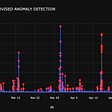# Mann Kendall Original Test

`import numpy as npimport pandas as pdimport pymannkendall as mkimport matplotlib.pyplot as pltimport statsmodels.api as sm%matplotlib inline# read Datsetdf= pd.read_csv("daily-total-female-births-CA.csv")print(df.head())df.plot(figsize=(12,8));plt.show()`
`from datetime import datetimedf['dated'] = pd.to_datetime(df['date'])df = df.set_index('dated')df.drop(['date'], axis=1, inplace=True)fig, ax = plt.subplots(figsize=(12, 8))sm.graphics.tsa.plot_acf(df, lags=20, ax=ax)plt.show()`
`import pymannkendall as mkimport matplotlib.pyplot as pltimport statsmodels.api as smprint(mk.original_test(df, alpha=0.05))`

# Hamed and Rao Modified MK Test

`shampoo_df = pd.read_csv('sales-of-shampoo-over-a-three-ye.csv', header=0)shampoo_df.dropna(inplace=True) # Droping the last row which contains NaNdef preprocess_data(df):    processed_df = df.rename(columns={        'Sales of shampoo over a three year period': 'shampoo_sales'    }).copy()    processed_df['Month'] = pd.to_datetime(processed_df.Month.apply(lambda val: '190'+val))    processed_df = processed_df.set_index('Month')    return processed_dfprocessed_df = preprocess_data(shampoo_df)# line plotprocessed_df.plot(figsize=(16, 7));plt.show()fig, ax = plt.subplots(figsize=(12, 8))sm.graphics.tsa.plot_acf(processed_df, lags=20, ax=ax);plt.show()`
`print (mk.hamed_rao_modification_test(processed_df))`
`print(mk.yue_wang_modification_test(processed_df))print(mk.trend_free_pre_whitening_modification_test(processed_df))print(mk.pre_whitening_modification_test(processed_df))`

# Seasonal MK Test

`Passenger_data = pd.read_csv("AirPassengers.csv",parse_dates=['Month'],index_col='Month')print(Passenger_data.head())Passenger_data.plot(figsize=(12,8))plt.show()`
`print(mk.seasonal_test(Passenger_data,period=12))`

# Output

--

--

--

## More from abhinaya rajaram

Data in Law

Love podcasts or audiobooks? Learn on the go with our new app.

## The power of data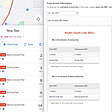## A Deep Dive into the Variance-Covariance Matrices of Classical Linear Regression Models## Explaining data science, AI, ML and deep learning to management — a presentation and a script —…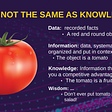## Basics of Data Preprocessing## Mindful Machines: Neuroscience & Critical Theory for Ethical AI## Five Open-Source Machine learning libraries worth checking out## Performing Analysis of Meteorological Data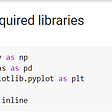Data in Law

## Soybean Crop Yield Prediction with ML Regression Techniques— Part 1: Tabular data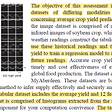## Dynamic Stochastic General Equilibrium (DSGE) Models — Free Course## Time Series Anomaly Detection with PyFBAD